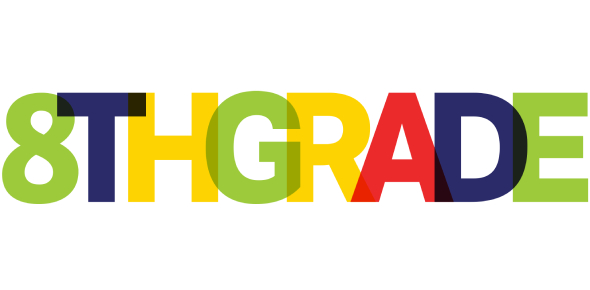# Pre Semester 2 Math Test For Grade 8 (Part 3) -finish

5 Questions | Total Attempts: 40Settings• 1.
21.Pak Amran bought 2 adult tickets  and 2 child tickets for a circus show for Rp 50.000         Pak Rosidy paid Rp 95.000  for 4 adult tickets and 3 child tickets for the same circus         Show . The linier equation system that represents this statement is …
• A.

A. 2x + y = 50.000     4x + 3y = 95.000

• B.

B. 2x + 2y = 50.000                                 3x + 4y = 95.000

• C.

C. 2x + 2y = 50.000     3x + 4y = 95.000

• D.

D. 2x + 2y = 50.000     4x + 3y = 95.000

• 2.
22.Given that 2x + 3y = 3   and  x + 2y = -2  and then the sum  of   x and y  is …
• A.

A.1

• B.

B.3

• C.

C.5

• D.

D.7

• 3.
IF DC IS A TANGENT , AD = 3 cm AND BC = 2 cm, AB = 13 cm WHAT IS DC LONG ?      . . . . cm
• A.

A.14

• B.

B.13

• C.

C.12

• D.

D.11

• 4.
BD = 18  cm      DC =  32  cm     So area and perimeter of  ABC are ...... Cm square and ... cm
• A.

a.  600 cm2  and 120 cm

• B.

b.  500 cm2  and 140 cm

• C.

c.  400 cm2  and 160 cm

• D.

d.  300 cm2  and 170 cm

• 5.
A swimming pool is 60 m in length and 40 cm in width. The depth of the sallowest end is 1 m and it goes slantwise to the deepest of  3 m.the volume of the water in swimming pool is ………litres.
• A.

A.4,400,000

• B.

B. 4,680,000

• C.

C. 4,780,000

• D.

D. 4,800,000

Related TopicsBack to top